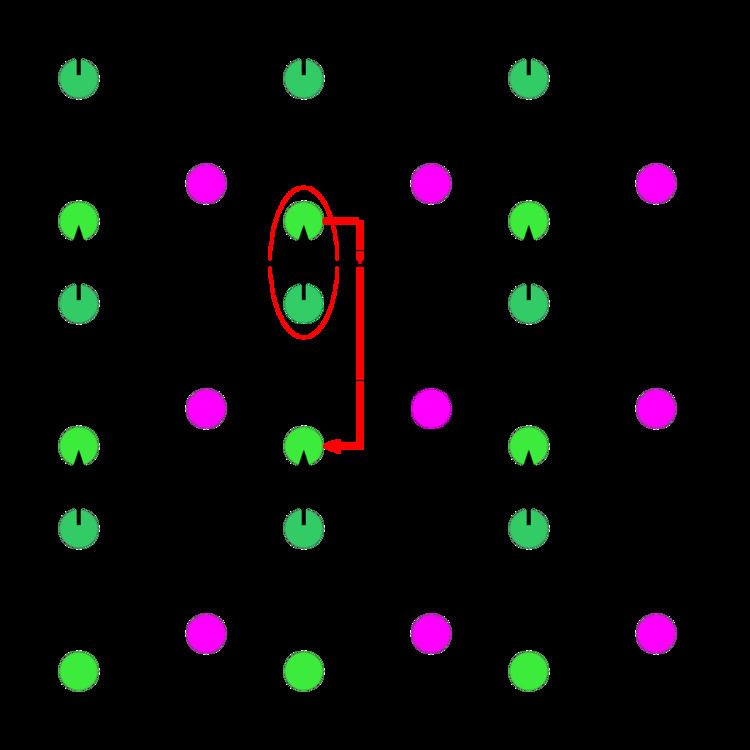# Equation of State Calculations by Fast Computing Machines

Updated on
Covid-19Equation of State Calculations by Fast Computing Machines is an article published by Nicholas Metropolis, Arianna W. Rosenbluth, Marshall N. Rosenbluth, Augusta H. Teller, and Edward Teller in the Journal of Chemical Physics in 1953. This paper proposed what became known as the Metropolis Monte Carlo algorithm, which forms the basis for Monte Carlo statistical mechanics simulations of atomic and molecular systems. The attribution of the method to Metropolis is unfortunate, as "Metropolis played no role in its development other than providing computer time". In fact, the theoretical work was done by Marshall N. Rosenbluth, who later gained renown as one of the greatest plasma physicists of the 20th century.

Monte Carlo methods are a class of computational algorithms that rely on repeated random sampling to compute their results. In statistical mechanics applications prior to the introduction of the Metropolis algorithm, the method consisted of generating a large number of random configurations of the system, computing the properties of interest (such as energy or density) for each configuration, and then producing a weighted average where the weight of each configuration is its Boltzmann factor, exp(−E/kT), where E is the energy, T is the temperature, and k is Boltzmann's constant. The key contribution of the Metropolis paper was the idea that

Instead of choosing configurations randomly, then weighting them with exp(−E/kT), we choose configurations with a probability exp(−E/kT) and weight them evenly.

This change makes the sampling focus on the low-energy configurations, which contribute the most to the Boltzmann average, resulting in improved convergence. To choose configurations with a probability exp(−E/kT) that can be weighed evenly, the authors devised the following algorithm: 1) each configuration is generated by a random move on the previous configuration and the new energy is computed; 2) if the new energy is lower, the move is always accepted; otherwise the move is accepted with a probability of exp(−ΔE/kT). When a move is rejected, the last accepted configuration is counted again for the statistical averages and is used as a base for the next attempted move.

The main topic of the article was the numerical calculation of the equation of state for a system of rigid spheres in two dimensions. Subsequent work generalized the method to three dimensions and to fluids using the Lennard-Jones potential. The simulations were done for a system of 224 particles; each simulation consisted of up to 48 cycles, where each cycle consisted of moving each particle once and took about three minutes of computer time using the MANIAC computer at Los Alamos National Lab.

To minimize surface effects, the authors introduced the use of periodic boundary conditions. This means that the simulated system is treated as a unit cell in a lattice, and when a particle moves out of the cell, it automatically comes in through the other side (making the system a topological torus).

According to a perspective published nearly fifty years later by William L. Jorgensen, "Metropolis et al. introduced the samplic method and periodic boundary conditions that remain at the heart of Monte Carlo statistical mechanics simulations of fluids. This was one of the major contributions to theoretical chemistry of the twentieth century." As of 2011, the article has been cited over 18,000 times.

In another perspective, it was said that although "the Metropolis algorithm began as a technique for attacking specific problems in numerical simulations of physical systems [...] later, the subject exploded as the scope of applications broadened in many surprising directions, including function minimization, computational geometry, and combinatorial counting. Today, topics related to the Metropolis algorithm constitute an entire field of computational science supported by a deep theory and having applications ranging from physical simulations to the foundations of computational complexity."

## References

Similar Topics
Guns of El Chupacabra
Alfredo Amezaga
Joseph M Petrick
Topics

 B i Link H2
 L